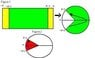# Riemman Integral: Path of \theta for \int^{\pi-\epsilon}_{-\pi+\epsilon}d\theta

• alejandrito29

#### alejandrito29

If the integral is $$\int^{\pi-\epsilon}_{-\pi+\epsilon}d\theta$$.

where $$\theta$$ is a angular coordinate.

In the riemman integral , i don't understand if tetha follows the path grenn in figure 1, or $$\theta$$ follows the path red in figure 2.

#### Attachments

•Dibujo.JPG
13.4 KB · Views: 343
The green path. The angle increases from $-\pi+ \epsilon$ to $\pi- \epsilon$ counterclockwise.